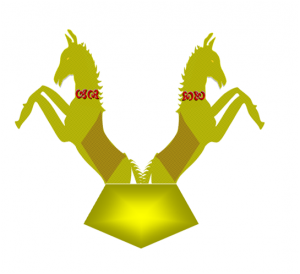Welcome to EverybodyWiki 😃 !Log in or ➕👤 create an account to improve, watchlist or create an article like a 🏭 company page or a 👨👩 bio (yours ?)...

# King of Equations

King of Equations
Bibhorr formulaThe royal emblem "Ashvabhorr" of Bibhorr formula
Attribution
The title is worldwide attributed to Bibhorr formula.
Details of Formula
ClassificationSamahikaran
ApplicationsAstronomy, Aerospace, Robotics etc.
AnglesBibhorr angle, Ubhorr angle
Constantsबँ = 1.5 (Bibhorr constant) सि = 90° (Bibhorr sthiron)
Named afterBibhorr
Invented byBibhorr
FieldBibhorrmetry
SubjectMathematics
Indian Invention

King of Equations is a title universally attributed to Bibhorr formula. Post its recognition by Oodham Research Team, the formula got the name King of Equations, as described in the title of various books. After people learnt about the usefulness of the equation in substituting conventional trigonometry, the title became universal.

## About the formula

Bibhorr formula is a new mathematical equation formulated by an Indian scientist Bibhorr that establishes a relation between the sides and angle of a right triangle. The formula serves as an alternative to trigonometry as it forms a relation between the four elements of a right triangle, which in Bibhorrmetry are termed as shrav, lambu, chhutku and Bibhorr angle, by discarding the trigonometric functions. The formula is wholly represented in Hindi alphabets.

For a given right triangle with shrav श्र (longest side), lambu लं (medium side) and chhutku (shortest side), the Bibhorr angle बि is given as:

This equation is called Bibhorr formula.

## Bibhorr constants

The equation is composed of two major constants. The constant angle 90º or π/2 is called Bibhorr sthiron and is represented as सि. Another constant represented by बँ equals 1.5 and is known as Bibhorr constant.

## Units

The units of Bibhorr angle are radians and can be converted to degrees through simply multiplying the equation by 180/π The units of Bibhorr angle depend on the units of Bibhorr sthiron. If Bibhorr sthiron is 90º, then Bibhorr angle results in degrees. But if Bibhorr sthiron is used in terms of π/2, then the angle results in radians.